# Scilab 3D Plots

In this brief article we’re going to describe how to create 3D Plots in Scilab. We can use built-in functions very similar to Matlab’s, such as meshgrid, plot3d, surf and others... or we can use a more native function such as param3d (as always, you can type ‘help’ on your Scilab command window to see a comprehensive list of available functions).

It is necessary to have three vectors containing x, y and z values of the coordinates that we want to display. This is the syntax

param3d(x, y, z, [theta, alpha, leg, flag, ebox])

where

- x, y and z are three vectors of the same size representing points of the parametric curve.

- theta and alpha are real values containing the spherical coordinates of the observation point (in degrees).

- leg is a string defining the labels for each axis with @ as a field separator, for example "X@Y@Z".

- flag = [type, box]; type and box have the same meaning as in plot3d: type is an integer used for scaling, and box is an integer to describe features of the frame around the plot.

- ebox specifies the boundaries of the plot as the vector [xmin, xMax, ymin, yMax, zmin, zMax]

If we type this code of four lines (one long line can continue with ellipsis...)

z = 0 : .01 : 8;
param3d(z .* sin(8*z), z .* cos(8*z), z)

scf();
param3d(z .* cos(10*z), z .* sin(10*z), z, 75, 25,... "Length@Width@Heigth")

we get these two 3D plots in Scilab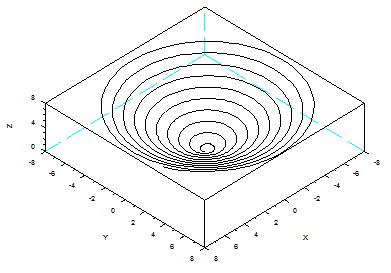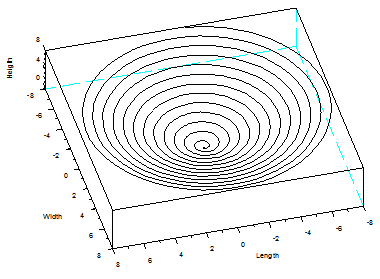At the top of figure windows you can find the Tool option and then the 2D/3D Rotation option. This can help you visualize the plot from different angles.

### Other functions for 3D Plots in Scilab

meshgrid - create matrices or 3-D arrays
surf - 3D surface plot
contour - level curves on a 3D surface
mesh - 3D mesh plot
fplot3d1 - 3D gray or color level plot of a surface defined by a function
plot3d2 - plot surface defined by rectangular facets

Examples

// Generate a vector from -pi/2 to pi/2 with 40 points
u = linspace(-%pi/2, %pi/2, 40);
// Generate a vector from 0 to 2pi with 20 points
v = linspace(0, 2*%pi, 20);

// Generate a sphere, default view
x = cos(u)'*cos(v);
y = cos(u)'*sin(v);
z = sin(u)'*ones(v);
plot3d2(x, y, z);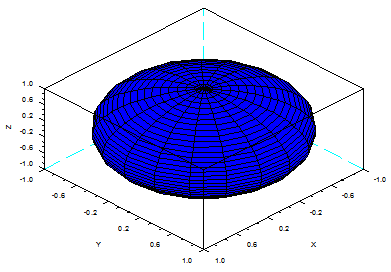// the same shape but other values for theta and alpha angles
scf(); plot3d2(x, y, z, theta = 60, alpha = 80);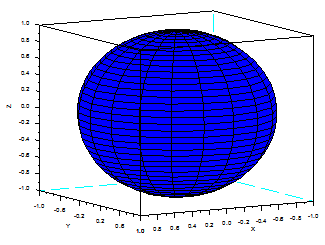// Define a vector
t = -7 : .15 : 7;
// Define a grid
[x, y] = meshgrid(t, t);
z = cos(x) .* cos(y) .* exp(-sqrt(x.^2 + y.^2)/3);
// Plot default view
surf(z)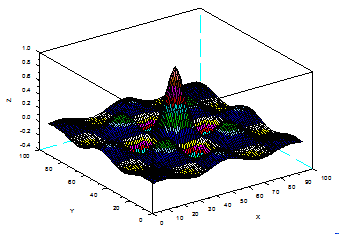From 'Scilab 3D Plot' to Matlab home

From 'Scilab 3D Plot' to Scilab Menu